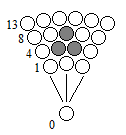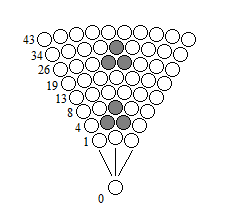# Evolution of x axis## Time dilation:

This dilation of time is the fact that when an object is formed on the high alternations of a spherical matrix, it will form less quickly than on the low alternations of the same matrix for one cycle.

Here is an example of a triangle-shaped object of a spherical matrix:

Legend of the distribution of time granted from the effective position:
po = origin point = 0,0001ns (position A)
→ = flow = ~0s (distance between A et B)
point(n) = dot matrix = 1ns (position B)(po–>point1–>po–>point2–>po–>point3–>) = line1 = ~3,0003ns
(po–>point4–>po–>point5–>po–>point5–>po–>point6–>po–>point6–>po–>point7–>) = line2 = ~6,0006ns
(po–>point8–>po–>point9–>po–>point10–>po–>point10–>po–>point11–>po–>point12–>) = line3 = ~6,0006ns
(po–>point13–>po–>point14–>po–>point15–>po–>point16–>po–>point17–>po–>point18–>) = line4 = ~6,0006ns

(line1 + line2 + line3 + line4) = cycle

cycle x frequency

From the first to the last point of the complete object, will be formed in about 9ns (~ 9,0009ns) for one cycle. The total refresh of the matrix ends in about 21ns (~ 21,0021ns) for one cycle.

Here if we continue this same matrix with additional lines to form a second object in the form of an identical triangle called object2, we have:(po–>point19–>po–>point20–>po–>point21–>po–>point22–>po–>point23–>po–>point24–>po–>point25–>) = line5 = ~7,0007ns
(po–>point26–>po–>point27–>po–>point28–>po–>point29–>po–>point29–>po–>point30–>po–>point30–>po–>point31–>po–>point32–>po–>point33–>) = line6 = ~10,0010ns
(po–>point34–>po–>point35–>po–>point36–>po–>point37–>po–>point38–>po–>point38–>po–>point39–>po–>point40–>po–>point41–>po–>point42–>) = line7 = ~10,0010ns
(po–>point43–>po–>point44–>po–>point45–>po–>point46–>po–>point47–>po–>point48–>po–>point49–>po–>point50–>po–>point51–>po–>point52–>) = line8 = ~10,0010ns

(line1 + line2 + line3 + line4 + line5 + line6 + line7 + line8) = cycle

cycle x frequency

The object2 is formed around 13ns (~ 13,0013ns). Either the same object is about 4ns longer on the high lines of the matrix, than its double in ~ 9,0009ns on the low lines. The object2 on the upper part of the matrix is ​​about 4ns older than its brother on the lower part. Because the effective position sweeps all the free positions end to end, it is logical to find in this case the extension of time when forming entirely identical objects. Subsequently we can understand that the movement of the object2 annihilates the scanning of the effective position, and thus the object2 is formed faster than if it remains motionless.

____ End ____